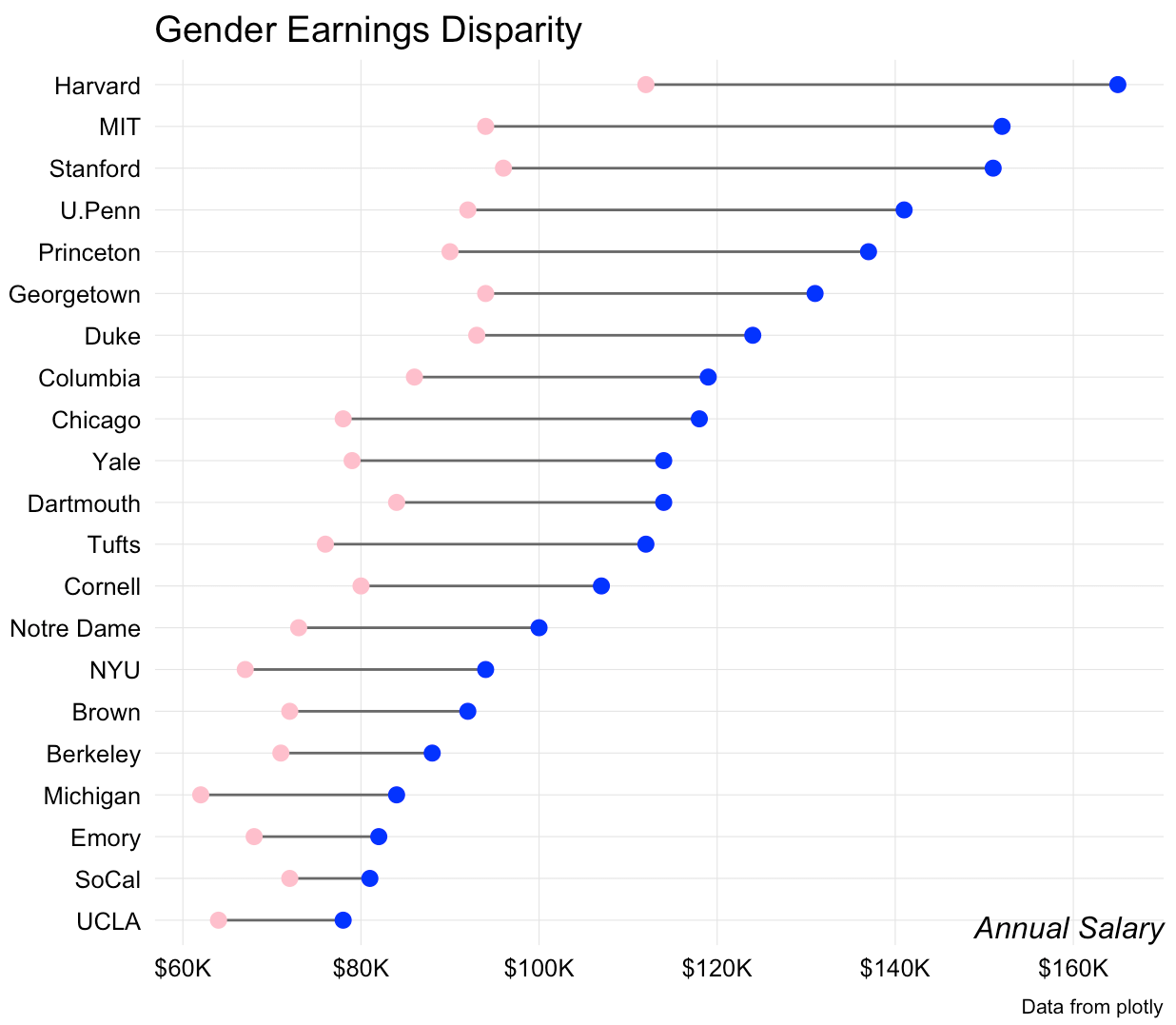# Beating lollipops into dumbbells

Shortly after I added lollipop charts to `ggalt` I had a few requests for a dumbbell geom. It wasn’t difficult to do modify the underlying lollipop `Geom`s to make a `geom_dumbbell()`. Here it is in action:

``````library(ggplot2)
library(ggalt) # devtools::install_github("hrbrmstr/ggalt")
library(dplyr)

# from: https://plot.ly/r/dumbbell-plots/
URL <- "https://raw.githubusercontent.com/plotly/datasets/master/school_earnings.csv"
fil <- basename(URL)

df <- arrange(df, desc(Men))
df <- mutate(df, School=factor(School, levels=rev(School)))

gg <- ggplot(df, aes(x=Women, xend=Men, y=School))
gg <- gg + geom_dumbbell(colour="#686868",
point.colour.l="#ffc0cb",
point.colour.r="#0000ff",
point.size.l=2.5,
point.size.r=2.5)
gg <- gg + scale_x_continuous(breaks=seq(60, 160, by=20),
labels=sprintf("\$%sK", comma(seq(60, 160, by=20))))
gg <- gg + labs(x="Annual Salary", y=NULL,
title="Gender Earnings Disparity",
caption="Data from plotly")
gg <- gg + theme_bw()
gg <- gg + theme(axis.ticks=element_blank())
gg <- gg + theme(panel.grid.minor=element_blank())
gg <- gg + theme(panel.border=element_blank())
gg <- gg + theme(axis.title.x=element_text(hjust=1, face="italic", margin=margin(t=-24)))
gg <- gg + theme(plot.caption=element_text(size=8, margin=margin(t=24)))
gg``````The API isn't locked in, so definitely file an issue if you want different or additional functionality. One issue I personally still have is how to identify the left/right points (blue is male and pink is female in this one).

### Working Out With Dumbbells

I thought folks might like to see behind the ggcurtain. It really only took the addition of two functions to `ggalt`: `geom_dumbbell()` (which you call directly) and `GeomDumbbell()` which acts behind the scenes.

There are a few additional, custom parameters to `geom_dumbbell()` and the mapped `stat` and `position` are hardcoded in the `layer` call. We also pass in these new parameters into the `params` list.

``````geom_dumbbell <- function(mapping = NULL, data = NULL, ...,
point.colour.l = NULL, point.size.l = NULL,
point.colour.r = NULL, point.size.r = NULL,
na.rm = FALSE, show.legend = NA, inherit.aes = TRUE) {

layer(
data = data,
mapping = mapping,
stat = "identity",
geom = GeomDumbbell,
position = "identity",
show.legend = show.legend,
inherit.aes = inherit.aes,
params = list(
na.rm = na.rm,
point.colour.l = point.colour.l,
point.size.l = point.size.l,
point.colour.r = point.colour.r,
point.size.r = point.size.r,
...
)
)
}``````

The exposed function eventually calls it's paired `Geom`. There we get to tell it what are required `aes` parameters and which ones aren't required, plus set some defaults.

We automagically add `yend` to the data in `setup_data()` (which gets called by the `ggplot2` API).

Then, in `draw_group()` we create additional `data.frame`s and return a list of three `Geom` layers (two points and one segment). Finally, we provide a default legend symbol.

``````GeomDumbbell <- ggproto("GeomDumbbell", Geom,
required_aes = c("x", "xend", "y"),
non_missing_aes = c("size", "shape",
"point.colour.l", "point.size.l",
"point.colour.r", "point.size.r"),
default_aes = aes(
shape = 19, colour = "black", size = 0.5, fill = NA,
alpha = NA, stroke = 0.5
),

setup_data = function(data, params) {
transform(data, yend = y)
},

draw_group = function(data, panel_scales, coord,
point.colour.l = NULL, point.size.l = NULL,
point.colour.r = NULL, point.size.r = NULL) {

points.l <- data
points.l\$colour <- point.colour.l %||% data\$colour
points.l\$size <- point.size.l %||% (data\$size * 2.5)

points.r <- data
points.r\$x <- points.r\$xend
points.r\$colour <- point.colour.r %||% data\$colour
points.r\$size <- point.size.r %||% (data\$size * 2.5)

gList(
ggplot2::GeomSegment\$draw_panel(data, panel_scales, coord),
ggplot2::GeomPoint\$draw_panel(points.l, panel_scales, coord),
ggplot2::GeomPoint\$draw_panel(points.r, panel_scales, coord)
)

},

draw_key = draw_key_point
)``````

In essence, this new geom saves calls to three additional `geom_`s, but does add more parameters, so it's not really clear if it saves much typing.

If you end up making anything interesting with `geom_dumbbell()` I encourage you to drop a note in the comments with a link.Amazon Author Page

## 9 Comments →Beating lollipops into dumbbells

1.Richard

The problem I see with this kind of lollipop charts is, that I cannot clearly grasp the endpoint. Is it the middle of the lollipop or is it the outer border? It may have some aesthetical appeal but a horizontal box plot without whiskers (or only whiskers with a vertical bar at the end |———————–| ) would be more readable.

1.hrbrmstr

Aye, I think for many (most) of them at least an arrow is necessary (it’s on the TODO).

2.Winfried

Would it be feasible to add unit tick marks to the lines between the markers?

1.hrbrmstr

Aye. That could be an option. How would you envision the API (i.e. parameter values) working?

1.Winfried

I need to think about it. It triggers more questions than answers. Clearly the unit you want to use depends on both the units used on the x-axis and the minimum and maximum difference (but what with differences close to zero).
How would a dumbbell chart look with a logarithmic scale on the x-axis? Would it be interpretable?

3.Bruno

I was wondering if is possible to change the shape of starting and ending in a dumbell graph.
This is because I want to highlight the variation across time so i think that an arrow could be more useful, for example:
1) a value has risen from 1 to 10 I would see something like ()=======>
2) a value has fallen from 10 to 1 I would see something like <======()
If is not possible probably is better to keep the ending point and skipping the beginning like

1) ========()
2) ()========

Do you think something like that is possible?

1.hrbrmstr
Thx for both using the pkg and taking the time to comment! Aye, I agree that this is a great idea and shld have time after I’m back from holiday to get it into the next rev of {ggalt}’s `geom_dumbbell()`.